#Function Repository Resource:

# FindFiniteModels

Find finite models consistent with the set of relations

Contributed by: Nikolay Murzin
 ResourceFunction["FindFiniteModels"][rels] finds models in form of multiplication tables consistent with the relations rels for each operator in rels, assuming each variable can have one of two values. ResourceFunction["FindFiniteModels"][rels,k] allows k≥2 values for each variable. ResourceFunction["FindFiniteModels"][rels,k,prop] returns a specified property prop of found models.

## Details and Options

The relations rels can be given in terms of operators in logical form with equalities/inequalities or string relations.
There are kka models for each operator of arity a.
Property prop may be one of following:
 "Association" returns an association with multiplication tables as values and indices as keys for each found model (default) "Indices" returns a list of indices for models
The following options can be given:
 "ExpressionPrune" the method to use "Parallelize" whether to run some operations in parallel limit the number of returned models
The following values can be given for the Method option:
 "ExpressionPrune" pruned search over relations converted to a conjunction of DNFs "BruteForce" a complete search over all possible models same as "ExpressionPrune"

## Examples

### Basic Examples (7)

Find binary models for a nullary operator:

 In:=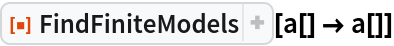Out=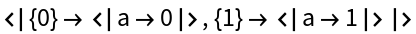Find ternary models for a nullary operator:

 In:=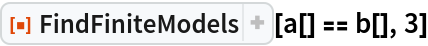Out=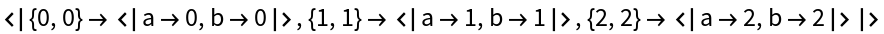Find binary models for unary operators:

 In:=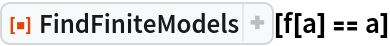Out=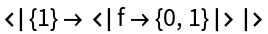In:=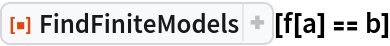Out=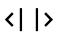In:=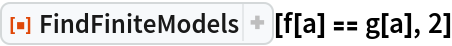Out=Find binary models for a binary operator:

 In:=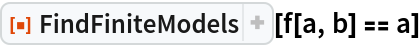Out=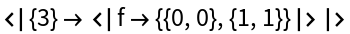Find ternary models for a binary operator:

 In:=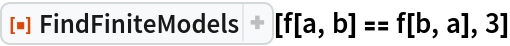Out=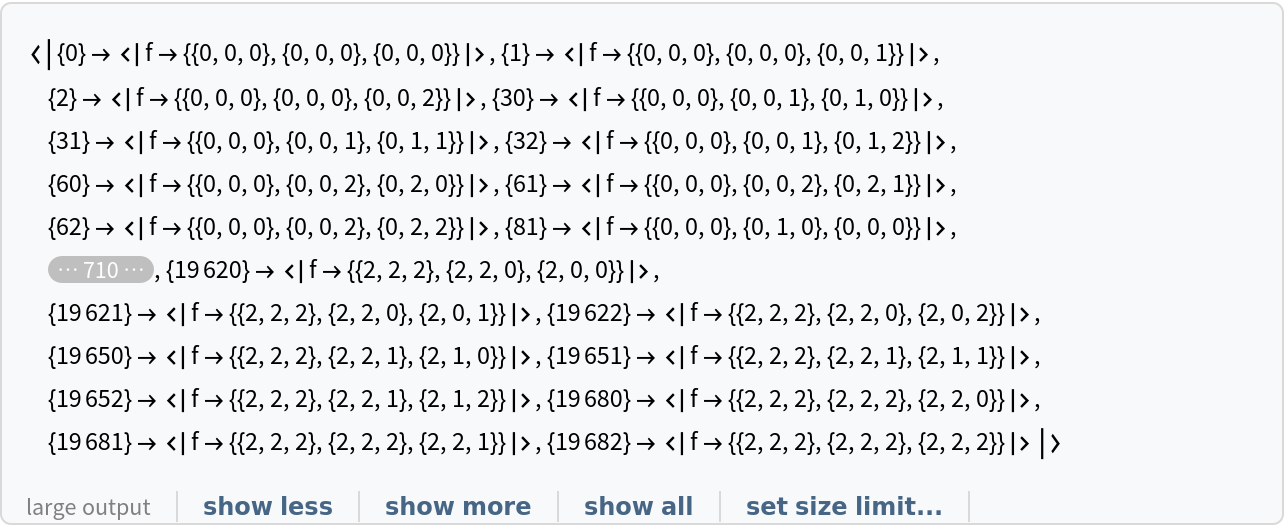Find binary models for a ternary operator:

 In:=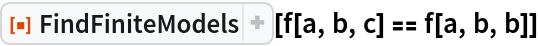Out=Include constants:

 In:=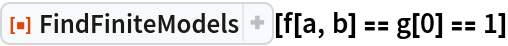Out=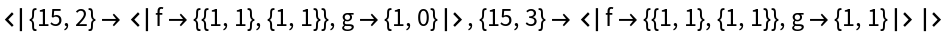### Scope (8)

In absence of operators, FindFiniteModels assumes all atoms are nullary operators:

 In:=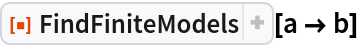Out=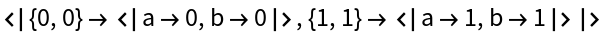Multiple operators with different arities are supported:

 In:=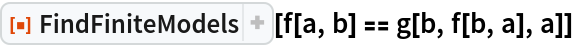Out=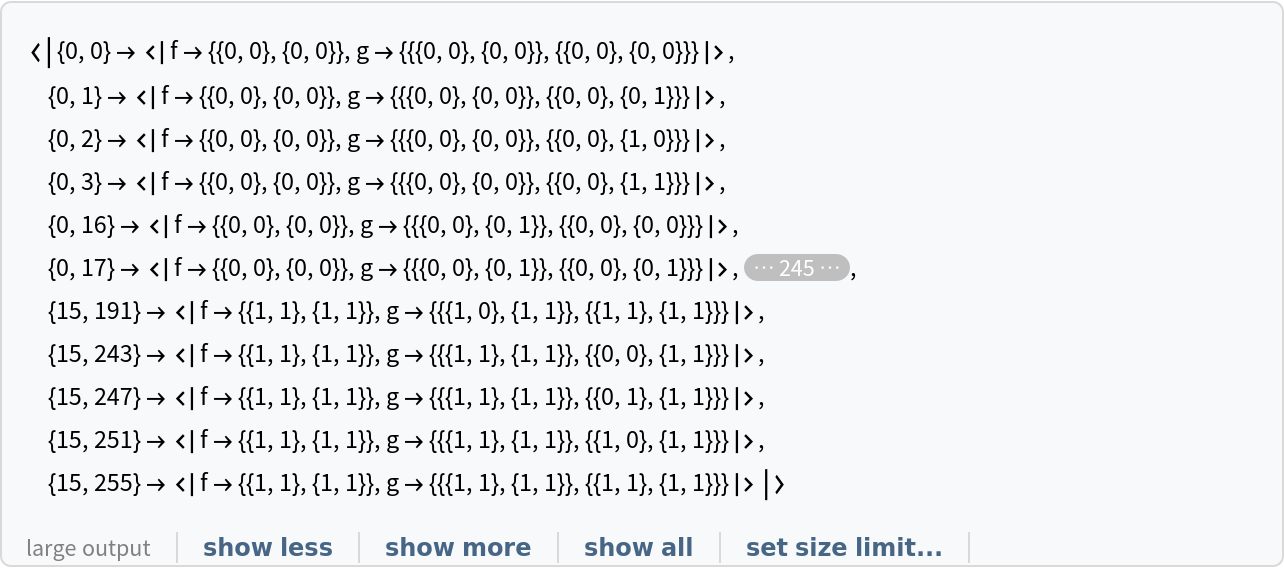Inequalities:

 In:=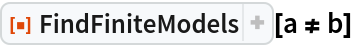Out=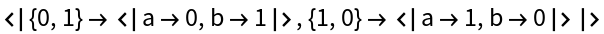In:=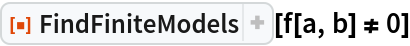Out=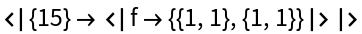In:=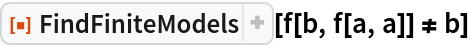Out=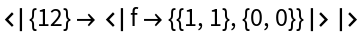Alternatives:

 In:=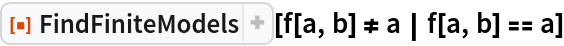Out=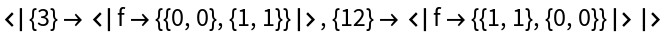Disjunctions:

 In:=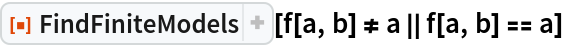Out=Quantifiers:

 In:=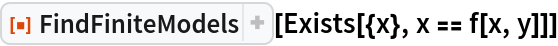Out=In:=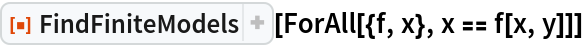Out=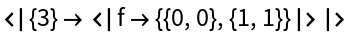In:=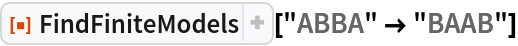Out=String relations form a monoid with binary associative operator ∘ (\[SmallCircle]) and nullary identity 1:

 In:=Out=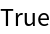### Options (3)

#### Method (1)

"BruteForce" method may be useful if a relation is very general so that enumerating models and finding a small subset of them returns faster:

 In:=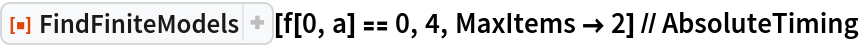Out=In:=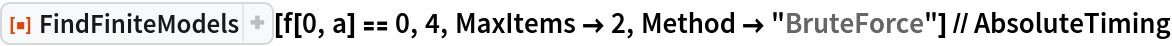Out=#### Parallelize (1)

Setting the option "Parallelize" to True may speed up computation:

 In:=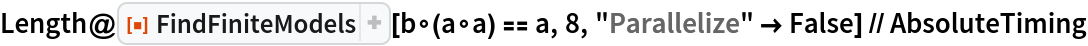Out=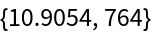In:=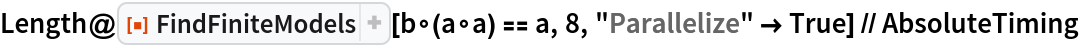Out=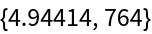In:=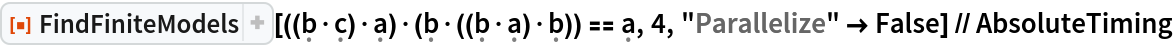Out=In:=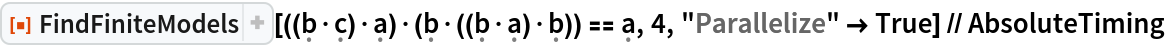Out=#### MaxItems (1)

Limiting a number of returned models reduces an amount of performed computation:

 In:=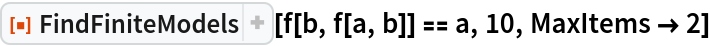Out=### Neat Examples (3)

Define functions to be used throughout this example:

 In:=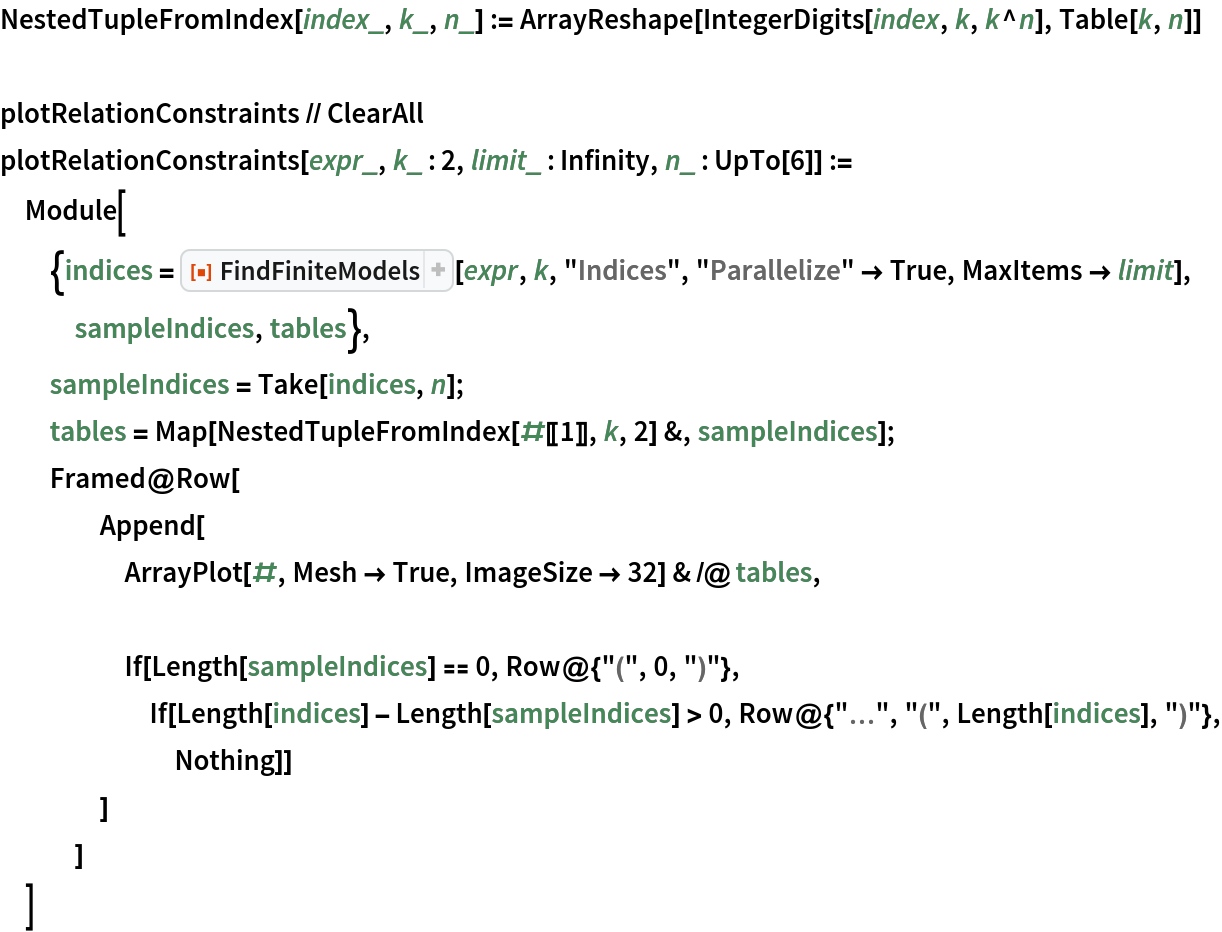Find models for abelian semigroup theory axioms:

 In:=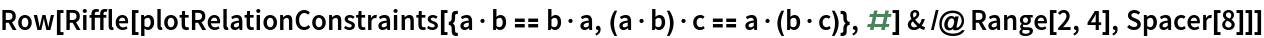Out=Reproduce and extend some NKS book examples:

 In:=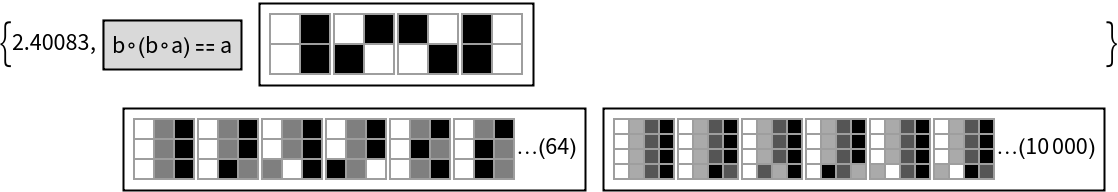In:=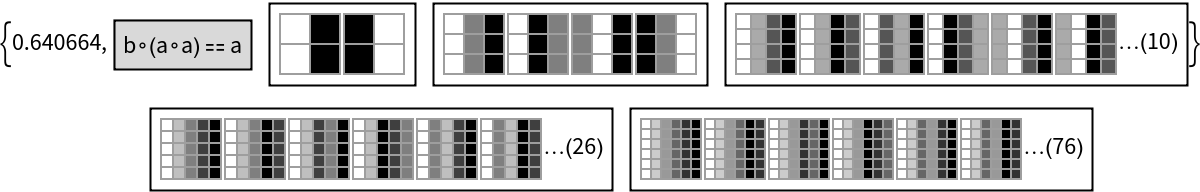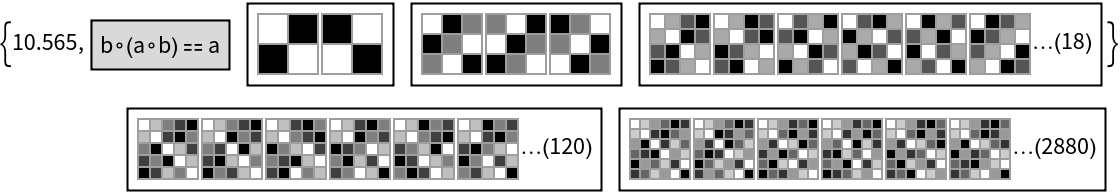In:=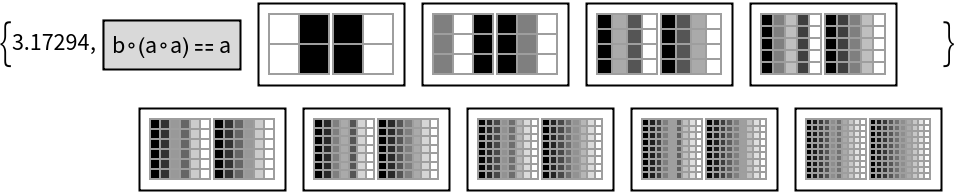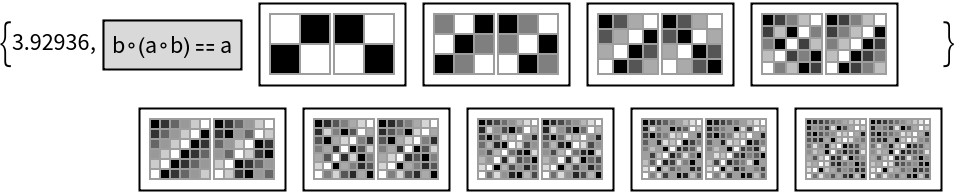In:=In:=Find models for the Wolfram axiom:

 In:=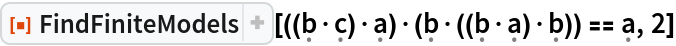Out=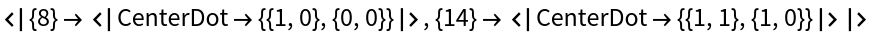In:=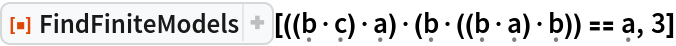Out=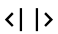In:=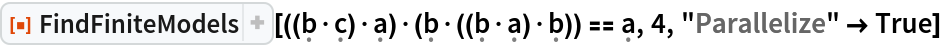Out=AxiomaticTheory examples:

 In:=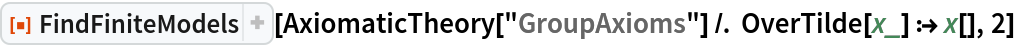Out=In:=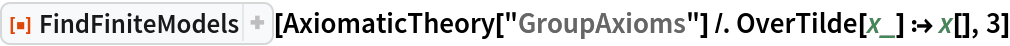Out=In:=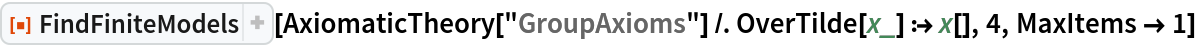Out=In:=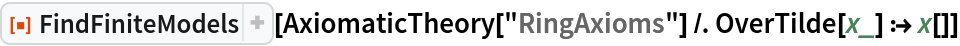Out=In:=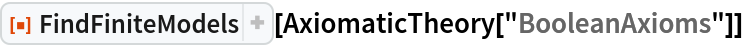Out=In:=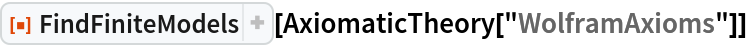Out=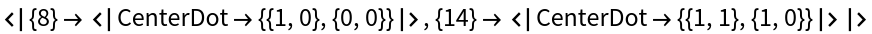In:=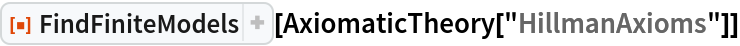Out=